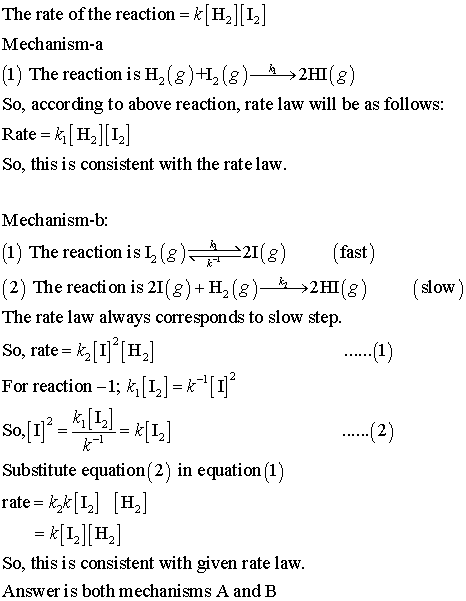# (a) which of these mechanisms are consistent with the observed rate law?

The reaction described by H2(g) + I2(g) yields
2HI(g) has an experimentally determined rate law of rate of reaction= k [H2] [I2] some proposed mechanisms for this reaction are: Mechanism a. 1. H2(g) + I2(g) yields (k1 on top of the arrow) 2HI
(g) (one step reaction) Mechanism b. (1) I2(g) equilbrium arrow (k1 on top of the arrow, and k-1 on
the bottom of the arrow ) 2I (g) (fast equlibrium
) (2) H2(g) + 2I(g) yields (k2 on top of the
arrow) 2HI(g) (slow) Mechanism c. (1) I2(g) equlibrium arrow (k1 on top of the
arrow, k-1 on the bottom of the arrow) 2I(g) (2) I(g) +H2(g) equlibrium arrow (k2 on the top and
k-2 on the bottom of the equilibrium arrow) yields
H2I(g) (Fast equlibrium) (3) H2I(g) yields ( k3 on top of the
arrow) HI(g) + I(g) (slow) a) which of these mechanisms are consistent with the observed
rate law? mechanism a mechanism b mechanism c b) in 1967 J.H Sullivan showed that this reaction was
dramtically catalyzed by light when the energy of the
light was sufficent to break the I-I bond in an I2 molecule. Which
mechanism(s) are consistent with both the rate law and this
additional observation? Mechanism a Mechanism b mechanism c

The rate of the reaction-kH2 I Mechanism-a (1) The reaction is H2(g)H, (e)-, 2HI(g) So, according to above reaction, rate law will be as follows Rate = k [H2]  So, thisis consistent with the rate law Mechanism-b (1) The reaction is L2 (21() (fast) (2) The reactionis 2I(g)+H2(g)»2HI(g) slow) The rate law always corresponds to slow step So, rate-ら| 1|-| H2 For reaction-1; kJa2-k-1 Substitute equation (2) in equation (1) rate -*-*II] [H] So, this is consistent with given rate law Answer is both mechanisms A and B